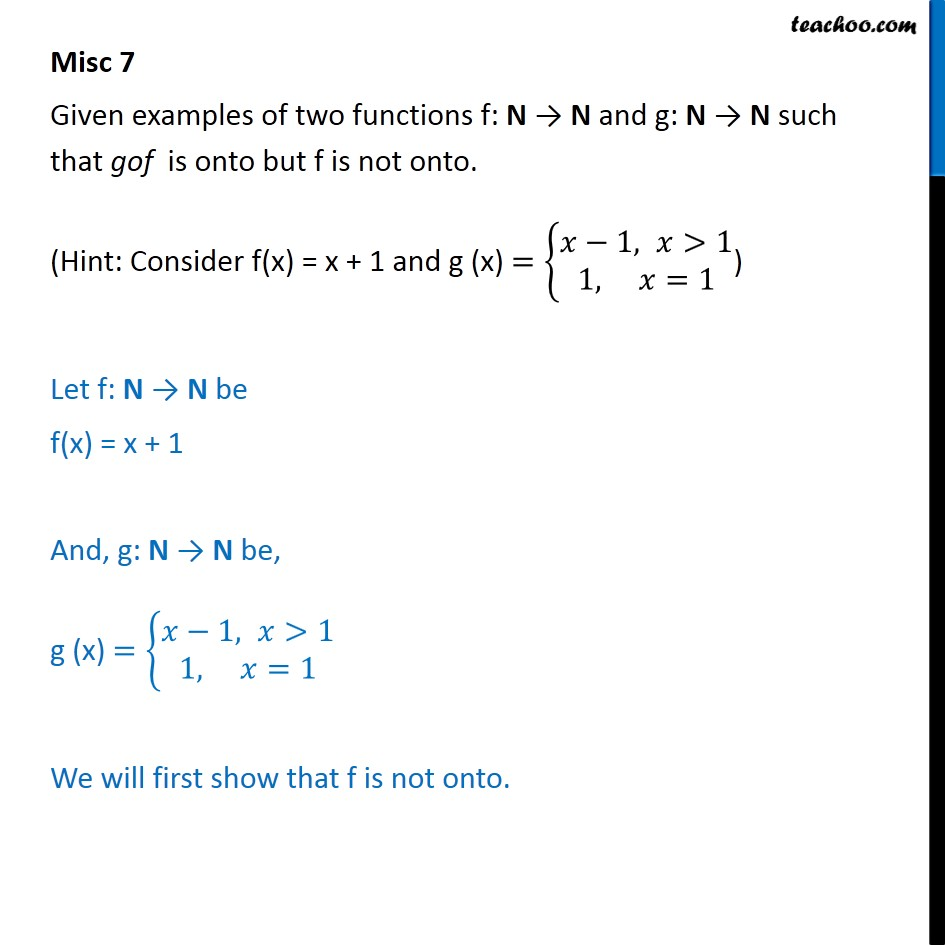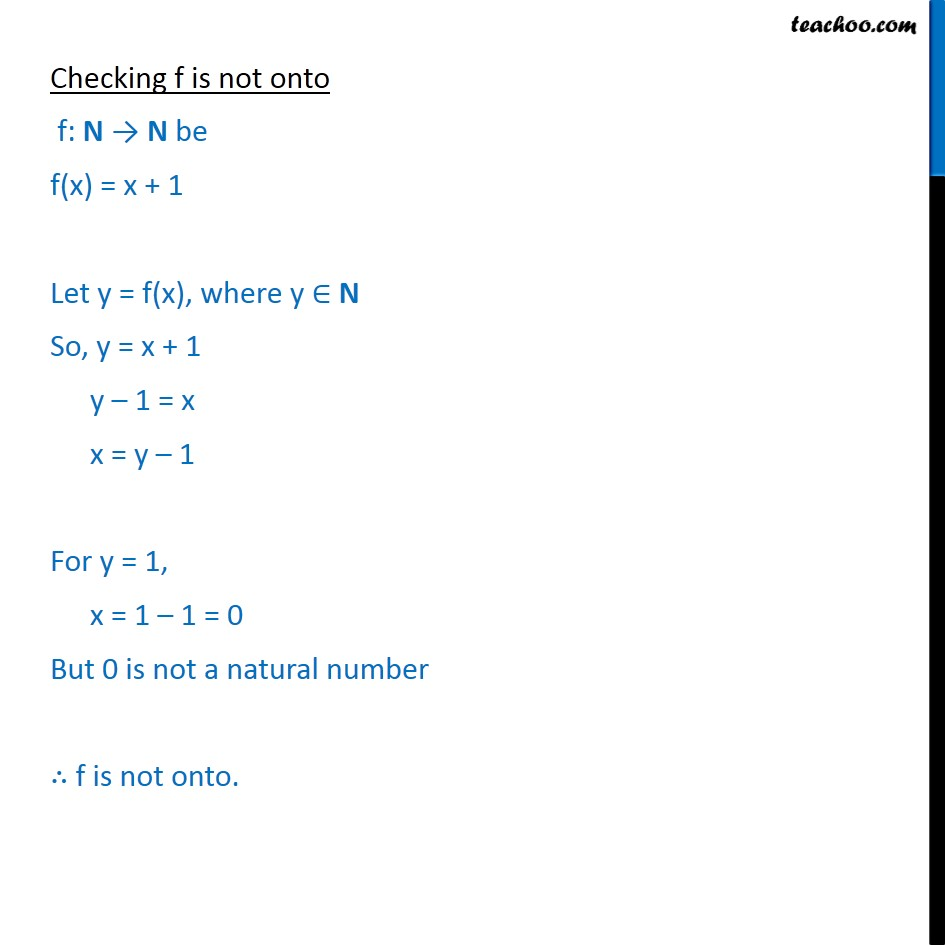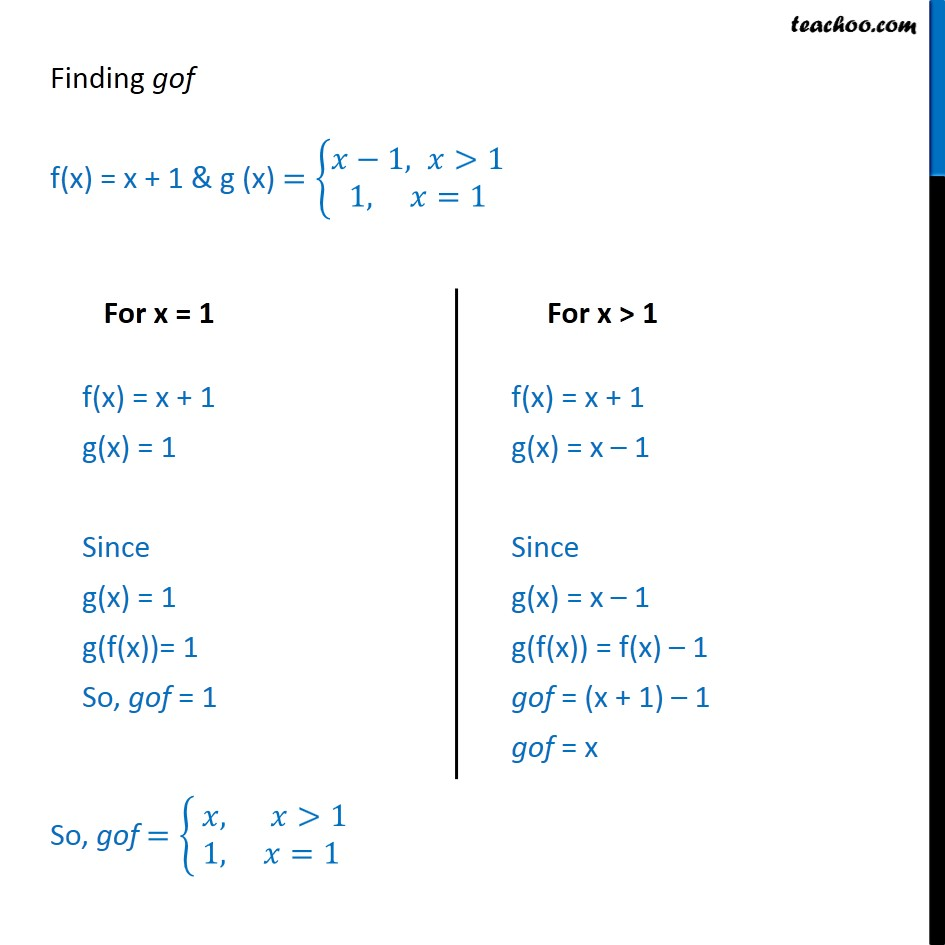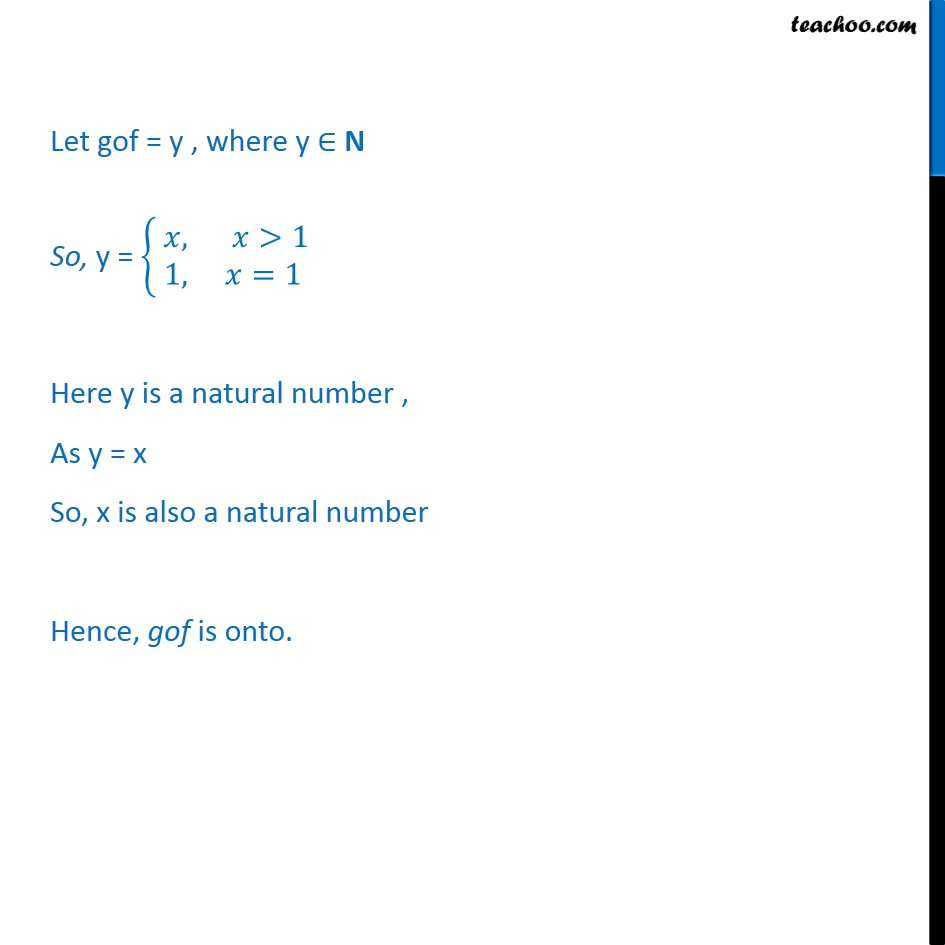Miscellaneous

Chapter 1 Class 12 Relation and Functions
Serial order wiseLearn in your speed, with individual attention - Teachoo Maths 1-on-1 Class

### Transcript

Question 5 Given examples of two functions f: N N and g: N N such that gof is onto but f is not onto. (Hint: Consider f(x) = x + 1 and g (x) = 1, >1 &1, =1 ) Let f: N N be f(x) = x + 1 And, g: N N be, g (x) = 1, >1 &1, =1 We will first show that f is not onto. Checking f is not onto f: N N be f(x) = x + 1 Let y = f(x), where y N So, y = x + 1 y 1 = x x = y 1 For y = 1, x = 1 1 = 0 But 0 is not a natural number f is not onto. Finding gof f(x) = x + 1 & g (x) = 1, >1 &1, =1 So, gof = , >1 1, =1 Let gof = y , where y N So, y = , >1 1, =1 Here y is a natural number , As y = x So, x is also a natural number Hence, gof is onto.